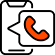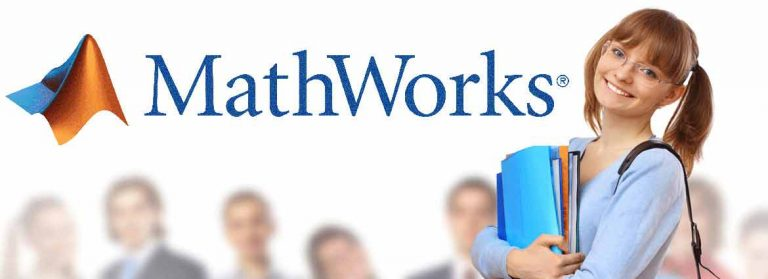02-6766 494 dukeuae@eim.aeHave Any Query?

### MATLAB FundamentalsCourse Purpose:
This  course provides a comprehensive introduction to the MATLAB technical computing environment. The course is intended for beginning users and those looking for a review. No prior programming experience or knowledge of MATLAB is assumed. Themes of data analysis, visualization, modeling, and programming are explored throughout the course. Topics include:

• Working with the MATLAB user interface
• Entering commands and creating variables
• Analyzing vectors and matrices
• Visualizing vector and matrix data
• Working with data files
• Working with data types
• Automating commands with scripts
• Writing programs with logic and flow control
• Writing functions

### Course Objective:

Working with the MATLAB User Interface

Objective: Become familiar with the main features of the MATLAB integrated design environment and its user interfaces. Get an overview of course themes.

• Plotting data
• Customizing plots
• Calculating statistics and best -fit line.
• Exporting graphics for use in other applications

Variables and Expressions

Objective:

Enter MATLAB commands, with an emphasis on creating variables, accessing and manipulating data in vector variables, and creating basic visualizations.

• Entering commands
• Creating numeric variables
• Creating character variables
• Making and annotating plots
• Getting help
• Accessing and modifying values in variables.

Analysis and Visualization with Vectors

Objective: Perform mathematical and statistical calculations with vectors. Use MATLAB syntax to perform calculations on whole data sets with a single command.

• Performing calculations with vectors
• Creating multiple plots

Automating Commands with Scripts

Objective:

Collect MATLAB commands into scripts for ease of reproduction and experimentation. Divide scripts into logical sections for development, maintenance, and publishing.

• Using the Command History
• Creating script files
• Running scripts
• Dividing code into sections
• Publishing scripts

Analysis and Visualization with Matrices

Objective:

Use matrices as mathematical objects or as collections of (vector) data. Understand the appropriate use of MATLAB syntax to distinguish between these applications.

• Creating and manipulating matrices
• Performing calculations with matrices
• Calculating statistics with matrix data
• Visualizing matrix data

Dates and Times

Objective:

Use variables to represent and manipulate dates and time durations.

Extract components of dates and durations as numeric variables.

• Representing dates and durations
• Performing calculations with dates and durations
• Plotting with dates
• Extracting numeric components of dates and durations

Tables of Data

Objective:

Import data as a MATLAB table. Work with data stored as a table.

• Storing data as a table
• Operating on tables
• Extracting data from tables
• Modifying table

Conditional Data Selection

Objective:

Extract and analyze subsets of data that satisfy given criteria.

• Logical operations and variables
• Finding and counting
• Logical indexing

Analyzing Data from Files

Objective:

Perform typical data analysis tasks in MATLAB, including importing data from file, preprocessing data, fitting a model to data, and creating a customized visualization of the model.

• Importing from spreadsheets and delimited text files
• Dealing with missing data
• Plotting functions
• Customizing plots

Flow Control

Objective:

Create flexible code that can interact with the user, make decisions, and adapt to different situations.

• Programming constructs
• User interaction
• Flow control
• Loops

Writing Functions

Objective:

Increase automation by encapsulating modular tasks as user-defined functions. Understand how MATLAB resolves references to files and variables. Use MATLAB development tools to find and correct problems with code.

• Creating functions
• Calling functions
• Setting the MATLAB path
• Debugging with the MATLAB Editor
• Using breakpoints
• Creating and using structures

Quick Enquiry

If you have any general course enquiries, please fill the form and get in touch today.

Call Us @